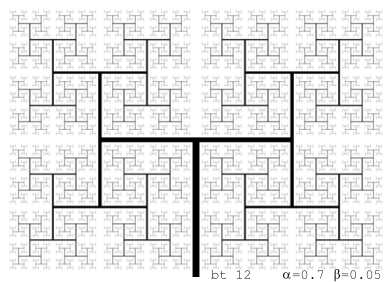Fractal antennaeFractals are good antennae. Fractal antennae are 25% more effective than the "rubber antennae", which has reigned supreme for mobile phones, cordless phones and walkie-talkies. Fractal antennea has a couple of advantages. They are well suited for wideband. And they do not need adjustment with electronic components, which makes them simpler and smaller.

In retrospective many advanced antenna designs has been approximations to fractals, but it was not until 1988 that fractals was used knowingly for designing antennae. Because that was when the ham radio enthusiast Nathan Cohen had to set up his system in his flat in central Boston. The lease stipulated no antennae on the outside of the building, so he had to be inventive. He had read Mandelbrots fractal book and got the idea to try out a fractal antenna. He cut out aluminium foil in the form of an inverse Koch curve and glued it to a piece of A4 paper. It worked amazingly well. Not until later did he realize how innovative he had been. In the time that followed he tested the antenna properties for other fractals, with good results, and then he founded Fractal Antenna Systems in 1995.To understand how antennae work you have to use Maxwells equations. That is not easy, and there is not yet a full understanding of why fractals work so well as antennae. But there is progress. In 1999 in the magazine Fractal Robert Hohlfeld and Nathan Cohen proved that for an antenna, to work well for all frequencies, it has to be symmetrical and self-similar.

1. symmetrical: means that the figure looks the same as its mirror image
2. self-similar: means that parts of the figure are small copies of the whole figure

These two properties are very common for fractals.

When I made my web-page about the Sierpinski fractals in 1998 I had no idea that they could be used for practical purposes. I think I can say that most of those, who read Mandelbrots pioneering fractal book in the 1980s, had no idea that fractals had any practical uses. Fractals was studied because they were interesting, beautiful mathematical objects in them selves.

Mathematics without practical uses turns unexpectedly into mathematics with practical uses. That has happened many times before.

An example. The ancient greeks was fascinated by prime numbers, and mathematicians since then was fascinated by prime numbers. But there was really no practical uses for the mathematics they developed, factorization, prime testing, big prime numbers... Then in the 1970s public key cryptography was invented. The algorithms that was invented then, which is in practical use today, uses just this mathematics.Another example. In the 1800s mathematicians, like Bernhard Riemann and Nizhny Lobachevsky, developed the non-euclidian geometry. It is, put simplistically, a geometry where straight lines can be bend. Really mathematically interesting, but noone could find practical uses for these geometries. More wellknown Albert Einstein developed in 1905-1915 the Relativity Theory. It was a long tough struggle for Einstein. Shortly after his first publication of the Relativity Theory, the mathematician Hermann Minkowski took it and gave it a simpler formulation, so it said that we live in a 4-dimensional non-euclidian universe. Had Einstein known about non-euclidian geometry he could have spared himself a lot of trouble. Today Minkowski's non-euclidian reformulation of Relativity Theory is the one that is used.It is a binary tree drawn so it is self-similar.

Construction:

 bt 0   = linesegment (0,0) (1,0) bt n   =   linesegment (0,0) (1,0)     ∪ (move (1,0))º(mul α)º(rot π/2) (bt n-1)     ∪ (move (1,0))º(mul α)º(rot -π/2) (bt n-1)

To avoid bt cutting into it self have:
α < 2-1/2 ≈ 0.7071

bt can easily be generalized to any number of dimensions (>1). For higher dimensions the α factor can be bigger, the inequality generalizes to:
α < 2-1/d

The picture above does not use line segments, but long thin rectangles. The length of the rectangles are the same as the line segment lengths l, the widths are β·l. To avoid the rectangles cutting into each other have:
2·α·( 2 - 1/(1-α2) ) > β

 http://www.h33.dk/fraktenne_index.en.html last changed 27.mar.2005 © 1997-2005 Bjørn Hee, mailto:webmaster@h33.dk